LemmaSC-parabola.mw

 > restart;

 > with( plots ):

 > with( plottools ):

 >

Shrinking Circle Problem

Enhanced Solution to the Original Shrinking Circle Problem

when the Fixed Curve is a Parabola

9 February 2007

 >

Setup the Geometry of the Problem

 > C  := a -> y^2 = a*x;          # fixed line

 > Cr := r -> x^2+y^2=r^2;        # shrinking circle

 > P  := r -> [ 0, r ];           # top of shrinking circle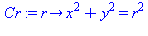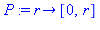>

 > plotP  := r -> plot( [P(r)], x=-1..1, style=point, symbol=circle, symbolsize=10, thickness=3, color=blue ):

 > plotC  := a -> implicitplot( C(a), x= -1..a, y=-a..a, color=pink, grid=[25,25] ):

 > plotCr := r -> implicitplot( Cr(r), x=-r..r, y=-r..r, color=cyan ):

 > P1 := (r,a) -> display( [plotP(r),plotC(a),plotCr(r)], view=[-r..max(r,a),DEFAULT], scaling=constrained,                        axes=normal, labels=["x","y"] ):

 > P1(1,2);

 >

The Point Q: The Intersection of C and C_r

These ellipses intersect in two points.

 > Intersection := [allvalues( solve( {C(a),Cr(r)}, {x,y} ) )] ;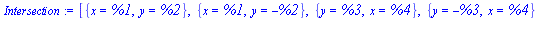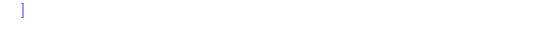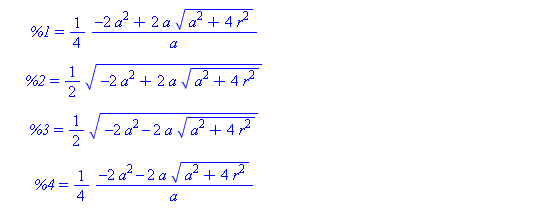Of the two points, we want the one in the first quadrant

 > X,Y := eval( [x,y], select( p->evalb( eval( [x,y], eval(p,[r=1.,a=2.]) )::[positive,positive] ), Intersection )[] )[]: x=X; y=Y;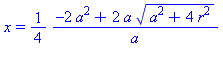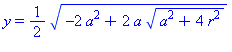>

This is the formula for the point Q.  .

 >

To construct the projection from the top of the shrinking circle through Q onto the y=0 plane,

 > Q := unapply( [ X, Y ], [r,a] ):

 >

 > plotQ := (r,a) -> plot( [Q(r,a)], color=gold, style=point, thickness=2 ):

 > P2 := (r,a) -> display( [plotP(r),plotC(a),plotCr(r),plotQ(r,a)],                         axes=normal, labels=["x","y"], scaling=constrained ):

 > P2(1,2);

 >

The Point R: The Intersection of line through PQ and the x-axis

For each angle theta, the lines passing through P and the point Q(theta) can be parameterized in terms of the (scaled) distance measured along this line.

 > LinePQ  := unapply( expand( (1-alpha)*P(r) + alpha*Q(r,a) ), [alpha,r,a] );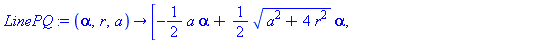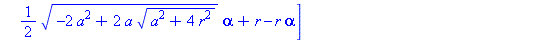>

The value of the parameter alpha when these lines hit the z=0 plane are given by

 > alpha0   := unapply( [simplify( solve( LinePQ(alpha,r,a)=0, alpha ) ) assuming a>0, r>0][],                     [r,a] );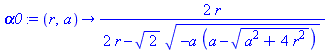>

Thus, the parametric representation of of the projected point, R, in the y=0 plane is

 > R := unapply( [simplify( LinePQ(alpha0(r,a),r,a) ) assuming a>0, r>0][], [r,a] );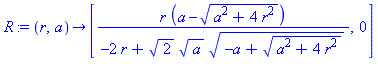>

This completes the constructions needed to put all of this together in one animation.

 > plotR := (r,a) -> plot( [P(r),R(r,a)], color=red, thickness=1 ):

 > P3 := (r,a) -> display( [P2(r,a),plotR(r,a)] ):

 >

These plots already illustrate the rapid convergence of every point on the curves R - except the one on the x-axis - to the origin (as r->0). Let's look at the parametric form of R. The three components are:

 > X,Y := R(r,a)[]: x=X; y=Y;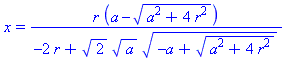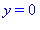>

The Limit as r -> 0

This limit is, once again, an indeterminate form that gives Maple some troubles

 > limit( X, r=0, right );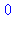>

until we give some extra information about the parameter

 > limit( X, r=0, right ) assuming a>0;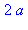>

We close with a different animation that shows this convergence.

 > plotR0 := a -> plot( [[2*a,0]], x=0..4*a, color=green, style=point, thickness=2 ):

 > animR := a -> animate( P3, [2-r,a], r=0..2, frames=30, numpoints=401, paraminfo=false, background=plotR0(a), view=[-2..max(2,2*a+2),DEFAULT] ):

 > animR(2);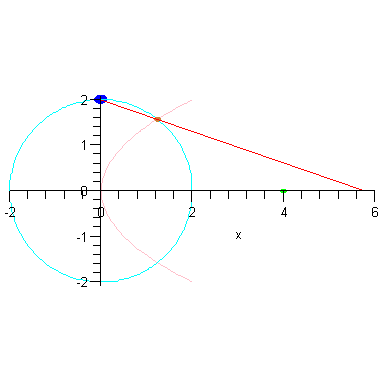>

 >